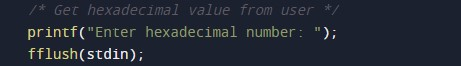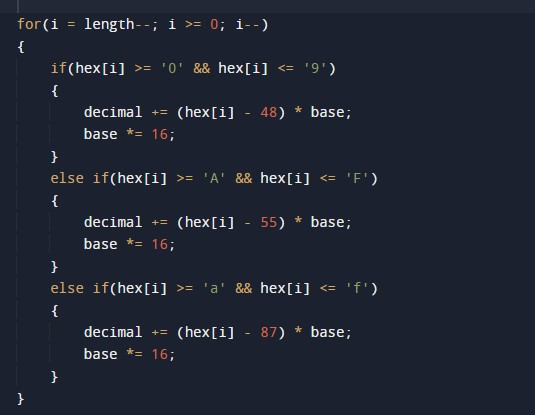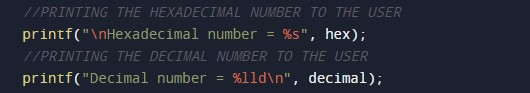C Program to Convert Hexadecimal to Decimal

In this tutorial you will learn about the C Program to Convert Hexadecimal to Decimal and its application with practical example.

C Program to Convert Hexadecimal to Decimal

In this tutorial, we will learn to create a C program that will Convert Hexadecimal to Decimal in C programming.

Prerequisites

Before starting with this tutorial we assume that you are best aware of the following C programming topics:

• Operators in C Programming.
• Basic Input and Output function in C Programming.
• Basic C programming.

Program to Convert Hexadecimal to Decimal:-

As we all know the c is a very powerful language. With the help of c programming language, we can make many programs. We cal perform many input-output operations using c programming. In today’s tutorial, we take the input in hexadecimal from the user and convert it into Decimal. With the help of c programming, we can perform many conversion operations.

With the help of this program, we can Convert Hexadecimal to Decimal.

Output:-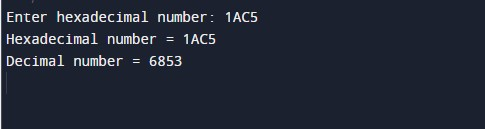In the above program, we have first initialized the required variable.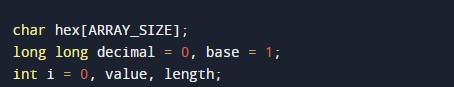• hex[] = it will hold the Hexa value for the program.
• i = it will hold the integer value.

Taking the input.